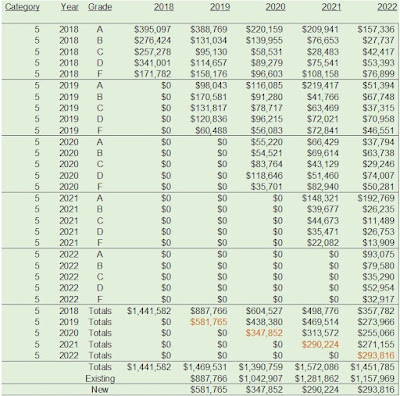## February 23, 2011

### Forecast Forensics + Digital Profiles: Converting Factors

Here's where the rubber meets the road, as they say!

Yesterday, we created four factors.  Today, we assign customers to one of sixteen Digital Profiles.

WARNING:  Geeky math alert ... feel free to skim if you don't like math!

We score each customer, by standardizing each variable (remember, yesterday we calculated the mean and standard deviation of each variable on a twelve-month basis), then we multiply the coefficients in the Component Score Coefficient Matrix by the standardized variables.  Here's the Component Score Coefficient Matrix:

At this point, I have four factors.

Next, if a factor has a value greater than or equal to zero, we assign a value equal to one, otherwise zero.  Once we do this, we combine four factors by two values each, yielding sixteen Digital Profiles.  Here's my SPSS code, if you're interested (the four factors are f1, f2, f3, and f4).

compute d1 = -0.0000.
compute d2 = -0.0000.
compute d3 = -0.0000.
compute d4 = -0.0000.
compute dp =  00.
if (f1 ge d1) and (f2 ge d2) and (f3 ge d3) and (f4 ge d4)  dp = 01.
if (f1 ge d1) and (f2 ge d2) and (f3 ge d3) and (f4 lt d4)  dp = 02.
if (f1 ge d1) and (f2 ge d2) and (f3 lt d3) and (f4 ge d4)  dp = 03.
if (f1 ge d1) and (f2 ge d2) and (f3 lt d3) and (f4 lt d4)  dp = 04.
if (f1 ge d1) and (f2 lt d2) and (f3 ge d3) and (f4 ge d4)  dp = 05.
if (f1 ge d1) and (f2 lt d2) and (f3 ge d3) and (f4 lt d4)  dp = 06.
if (f1 ge d1) and (f2 lt d2) and (f3 lt d3) and (f4 ge d4)  dp = 07.
if (f1 ge d1) and (f2 lt d2) and (f3 lt d3) and (f4 lt d4)  dp = 08.
if (f1 lt d1) and (f2 ge d2) and (f3 ge d3) and (f4 ge d4)  dp = 09.
if (f1 lt d1) and (f2 ge d2) and (f3 ge d3) and (f4 lt d4)  dp = 10.
if (f1 lt d1) and (f2 ge d2) and (f3 lt d3) and (f4 ge d4)  dp = 11.
if (f1 lt d1) and (f2 ge d2) and (f3 lt d3) and (f4 lt d4)  dp = 12.
if (f1 lt d1) and (f2 lt d2) and (f3 ge d3) and (f4 ge d4)  dp = 13.
if (f1 lt d1) and (f2 lt d2) and (f3 ge d3) and (f4 lt d4)  dp = 14.
if (f1 lt d1) and (f2 lt d2) and (f3 lt d3) and (f4 ge d4)  dp = 15.

if (f1 lt d1) and (f2 lt d2) and (f3 lt d3) and (f4 lt d4)  dp = 16.

With this logic ... and the instructions from above, I have sixteen Digital Profiles.

Next Week:  We describe each of the sixteen Digital Profiles that will be used in our Forecast Forensics analysis!  Contact me if you'd like to have your own customized Forecast Forensics / Digital Profiles analysis.